# Analysis of Possible Risks in Aviation Safety Issues Associated with the Massive Introduction of Unmanned Aerial Systems

Salwa FEZAI1*, Racha NEFZI2 and Brahim BEN-BEYA2

1Physics Department, Samtah University College, Jazan University, Kingdom of Saudi Arabia

2Laboratory of Physics of Fluids, Physics Department, Faculty of Science of Tunis, University of Tunis El-Manar, 2092 El-Manar 2, Tunis, Tunisia

Received Date: December 21, 2020;  Published Date: January 07, 2021

#### Abstract

The flow around three staggered square cylinders at two different triangular arrangements has been numerically analyzed in the present work. The calculations are carried out for several values of Reynolds numbers ranging from 1 to 110. Three different states of flow are found in this study by systematically varying Re. The critical Reynolds number is determined for both two arrangements. It is clear that the point of bifurcation is strongly influenced by the type of the type of the triangular arrangement because both arrangements generate a large reduction in the value of the critical Reynolds number. The unsteady periodic wake is characterized by the Strouhal number, which varies with the Reynolds number and also the arrangement of the three cylinders. Hence, the values of vortex shedding frequencies are calculated for both triangular arrangements. Furthermore, the drag and lift coefficients of three staggered squares cylinders in different triangular arrangements are determined.

Keywords:Staggered square cylinders; Flow regimes; Triangular arrangement; Strouhal number; Numerical simulation; Critical Reynolds number; Lift and drag coefficients

Nomenclature:

Re - Reynolds number

l - channel width

h - channel height

(u, v) - velocity components, m s-1

x, y - dimensionless coordinates

St - Strouhal number

CD - drag coefficient

CL - lift coefficient

P - dimensionless pressure

Φ - generic variable

Greek symbols

ρ - density of fluid, kg m-3

ν - kinematic viscosity, m2s-1

Subscripts

Max, Min - maximum, minimum

c - Critical

#### Introduction

The examination of flow structures around staggered cylinders at different arrangements is important because many issues related to aerodynamics are placed in category of these types of flow and in many applications. High-rise buildings, bridge piers, chimneys, wind tunnel, cooling towers are examples of these applications. However, three or more square cylinders make the problem complicated due to the interaction between shears layers and shedding vortices in the wake region. For this reason, many investigations on the flow around cylinders with variety of arrangements have been done. Indeed, the wake development behind these configurations depends on Reynolds number, spacing between obstacles and the structure of arrangement. Thus, it is important to understand the dramatic change in the wake structure. Therefore, many numerical investigations are available related to the characteristics of flow around two or more bodies in different arrangements. For better understanding of the phenomenon of Hop bifurcation, one can refer to the investigation of Yang and Zebib . They showed that when the Reynolds number of about 20, an absolutely unstable region begins to form, and grows more and more with Re. Furthermore, they deduced that the critical Reynolds number corresponds to a state where all wake is absolutely unstable.

Fezai et al.  also examined the transition from a symmetric flow state to the periodic state. They analyzed the vortex at different arrangements of the two shapes. The analysis of the flow evolution shows that with increasing Re beyond a certain critical value, the flow becomes unstable and undergoes a bifurcation. Therefore, they observed that the transition to unsteady regime is performed by a Hopf bifurcation.

The appearance criteria of different regimes such as crawling scheme, the steady and unsteady with releases of vortices strongly depends on the Reynolds number, so this conclusion was drawn by Berrone et al. . They also found that the results of numerical studies strongly depend on the choice of the mesh refinement, the passage of time and the domain size. This is well confirmed by the work of Noack and Eckelmann . They found that for all Reynolds less than 54 the flow is stable, while the periodicity appears for 54

The transitions in the wake of a square cylinder were also investigated by Gera et al. . They found that the flow around a single square cylinder remains steady up to Re = 50 and instability occurs between Re = 50 and 55. After this range, flow becomes completely unsteady. Similar observations were reported in the numerical work of Kelkar and Patankar . They concluded that the point of instability is between Re = 50 and 60 and they computed the value of the critical Reynolds number having a value Rec=53.

Lankadasu and Vengadesan  analyzed numerically incom pressible linear shear flow across a square cylinder. They found that the critical Reynolds number is reduced with increasing shear. Their results show that the mean drag coefficient decreases either with increasing shear for a particular Reynolds number or with increasing Reynolds number for a particular shear parameter.

In the work of Cheng et al. , it was reported that that the vortex shedding and wake development behind the square cylinder are significantly dependent on both the magnitude of the shear rate and the Reynolds number. Indeed, they found that vortex shedding disappeared for large shear parameters. The frequency of vortex shedding decreases as the shear parameter increases. Also, the drag coefficient tends to decrease with increasing shear parameter.

Mukhopadhay et al.  analyzed the structure of a flow around a square obstacle for different Reynolds number and for different positions of the obstacle. These researchers analyzed the effect of blockage ratio on the variation of Strouhal number based on the Reynolds number. In all cases, the Strouhal value increases with the blocking ratio and undergoes a slight change with increasing Re. Consequently, they determined the critical Reynolds number from which the flow becomes periodic and they concluded that the frequency starts at Re = 87 for a blockage ratio B/H = 0.25.

When two cylinders are placed in line, the flow characteristics could be substantially different from those of single cylinder due to interactions of the wakes of the two cylinders. Vikram et al.  examined the flow around two tandem square cylinders. They reported that the vortex shedding frequency was suppressed due to introduction of second square cylinder.

Abbasi and Islam  simulated wake interactions of a flow around two square cylinders which are placed in line with a fixed space ratio equal to 3.5. They concluded that the unsteady regime appears when the Reynolds number reaches the value 55.

Recently, Burattini and Agrawal  analyzed the flow around two square cylinders at a Reynolds number of 73. They reported different ranges of the wake flow regimes.

Rao et al.  investigated the effect of the space ratio on the flow around two square cylinders arranged side by side. They found that the frequency of vortex shedding is different in two wakes. The upper frequency is smaller than the lower frequency for small rations (s<1.4). However, when the space rations increase, the frequency of vortex shedding is almost equal in two wakes. They analyzed the influence of the space ration and Reynolds number on the drag and lift force. The difference of time-averaged drag and lift coefficients of the cylinders decreases with the increase in space ratios. When s=2.0 and 2.5, the curves for the time averaged drag and lift coefficient with different Reynolds numbers are smooth. Also, they reported that when s=1.5 and 1.8, the curves are smooth under Re<140, but that will be fluctuant under Re>140 because of the nonlinear interaction between the wakes and the instability of flow becomes stronger with the increase in Reynolds numbers.

Adeeb et al.  investigated computationally the wake flow of two square cylinders by varying the corner radius to understand the effect of the gap spacing’s role and the wake flow pattern at Re = 100. They found that the flow characteristics and vortex shedding depend significantly on the corner radius and gap spacing. They concluded that a square cylinder exhibited the maximum average drag value and the inverse was observed in the case of a circular cylinder. Furthermore, aerodynamic forces were reduced by rounding the corner radius.

Aboueian and Sohankar  examined the effect of the gap spacing on the flow over two square cylinders in staggered arrangement at Re=150. By changing the gap spacing between cylinders, five different flow regimes are identified and classified.

Investigations of flow characteristics of a row of square cylinders arranged in side-by- side configuration also attracted a considerable amount of interest in the past. Abbasi et al.  have conducted an investigation on the transition in flow states around two-, three- and four-inline square cylinders the effect of Reynolds numbers at two different gap spacing g = 2 and 5. Their results show that at g = 2, the range of Re for each flow state decreases by increasing the number of cylinders in the array, while at g = 5, they observed the opposite trend. Hetz et al.  have also found that the Strouhal number of five inline circular cylinders increases with increment in g. Manzoor et al.  reported that, at some spacing values, the downstream cylinders experience larger drag force as compared to upstream cylinders due to turbulence effects in flow even at low Re values. Liu  examined the dependence of flow induced forces on g and found that the drag coefficient (CD) of cylinders changes abruptly in the range between g= 3.5 and 4 due to existence of critical spacing value in this range.

Also, Zheng et al.  examined the effect of gap ratios ranging from 1.5 to 7 and two incidence angle ɵ= 0° and 180° on flow past three circular cylinders arranged in an equilateral triangular arrangement. They found that the proximity interference dominated the flow interference among three cylinders at small gap spacing g. At intermediate g, the gap flow became intense, weakening the proximity interference, while the impact of the proximity effect became very weak and wake effects dominate the flow interference among three cylinders at sufficiently large gap spacing. They also reported that for small g the fluctuating forces on downstream cylinders are very small and far less than those on a single cylinder due to the proximity effect.

Yang et al.  analyzed numerically the flow pattern and vortex suppression regions for three circular cylinders with two different staggered arrangements. In the first arrangement a primary cylinder was placed in front of downstream two side by side cylinders and in the second arrangement a primary cylinder was placed in the wake of upstream two side by side cylinders. Their results show that in the first arrangement, the vortex shedding behind the primary cylinder was suppressed by the downstream two side by side cylinders at 100≤Re≤200. They also reported that in the second arrangement the vortex shedding of the primary cylinder was suppressed by the upstream two side by side cylinders at 103≤Re≤175.

We can also mention the work of Yang et al. . They arranged three stationary circular cylinders in such a way that one cylinder was placed in front of two side by side cylinders and investigated the effect of the gap ratio in the range from g=1-10 for Re=200. They observed steady flow region at 1≤g≤1.2 and 2.5 ≤g≤3.1 and unsteady flow region at 1.3≤g≤2.4 and 3.2≤g≤10. They also found regular drag and lift coefficient in steady flow region which became irregular in unsteady flow region.

Bao et al.  investigated the flow past an inline array of six square cylinders at Re = 100 and ranging from 1.5 to 15. They observed six different kinds of flow patterns: steady wake, non-fully developed vortex street in single row and double-row, fully developed vortex street in double-row, fully developed vortex street in partially recovered single-row, and fully developed multiple vortex streets. They also observed that the first and second cylinder behave similar to the two inline cylinders configuration in terms of aerodynamic force coefficients while the other four cylinders experience periodic variation of forces with the increase in gap spacing.

The literature survey shows that not much attention has been paid to the flow around square cylinders in triangular arrangement. This type of flow has not been examined yet for a wide range of Reynolds number and global parameters are not fully investigated. In the numerical work of Rahman et al. , the value of the critical Reynolds number, which characterizes the bifurcation point from steady state to unsteady sate, has not been determined and they have limited their work to a single value the Reynolds number which has been set at 150. However, this limitation is not there in this work.

Therefore, in this paper, we will focus on the flow around three square cylinders at two different types of triangular arrangements. The motivation of current study is to determine the exact value of the critical Reynolds number for both arrangements and analyze the effect of changing the arrangement of the three square cylinders on the vortex shedding and on the drag and lift forces in order to analyze the flow structure around two different arrangement at steady, transitional and unsteady states.

The first task is essentially composed of six parts. Firstly, we present the configuration of the physical problem. The second part is devoted to presentation of equations and boundary conditions. The numerical method used for solving the system of Navier-Stokes equations in its dimensionless form in the case of a two-dimensional (2D) incompressible flow is presented in the third part. The fourth part is dedicated to the validation of the numerical in-house code used and followed finally by results and discussions. The fifth part is consecrated for results and discussions. Finally, we will draw conclusions from this study in a final part.

#### Physical Problem

The geometry of the problem of this particular study consists of a two-dimensional flow of a Newtonian incompressible fluid around three staggered square cylinders situated in a channel having a width l = 50H and a height h = 8H as sketched in Figure 1.

In the present study, two configurations will be considered by varying the arrangement of the three cylinders C1, C2 and C3. For the first arrangement, the configuration is composed of three cylinders C11, C21 and C31 as shown in the figure, but in the second configuration the arrangement of the three cylinders will be reversed from the first configuration. In Figure 2 we have schematized the two arrangements that we will study and analyze.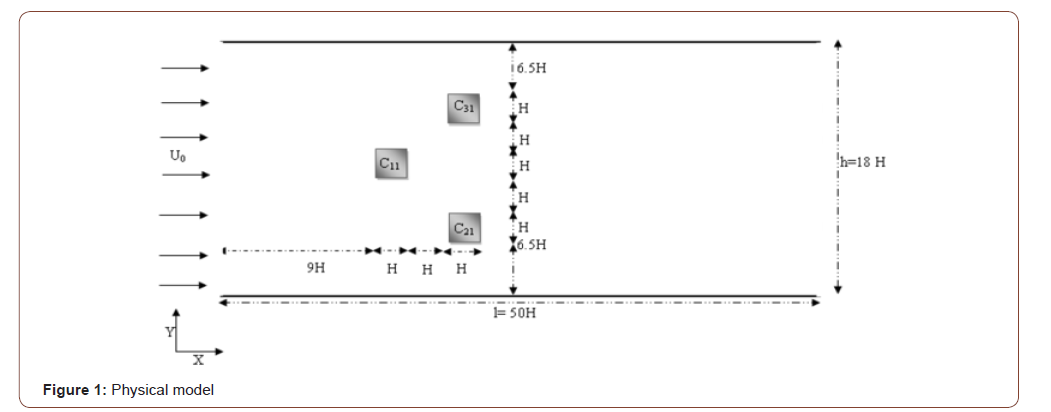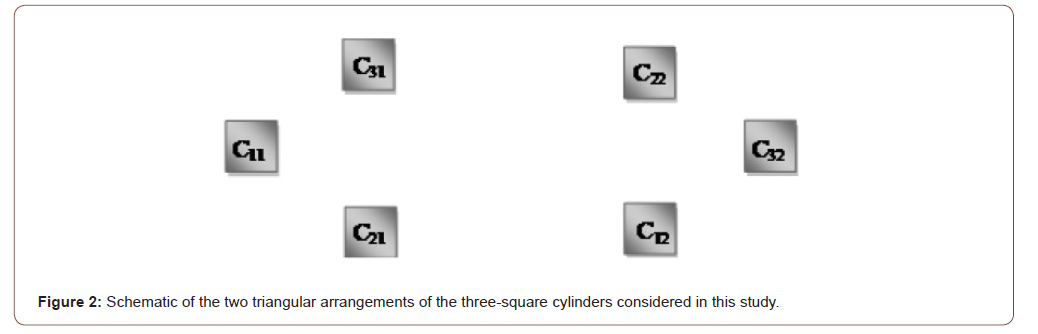##### Governing flow equations

The physical problem is governed by the Navier–Stokes equations for two-dimensional incompressible fluid flow. The continuity and momentum equations are written in dimensionless form expressed as follows:

Generally, a dimensionless writing of the Navier-Stokes equations shows the usual dimensionless numbers. These parameters characterize the similarity structure and stability of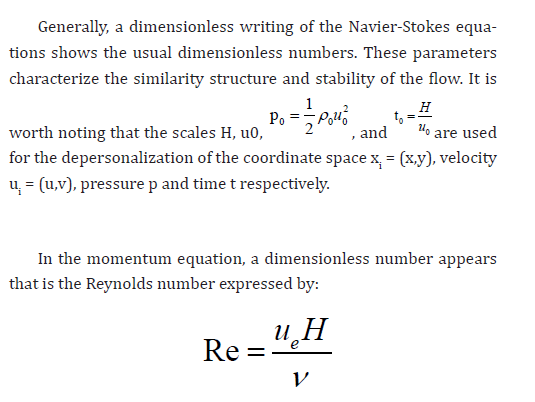Where ue= u0 is the velocity upstream, H is the side of the barrier ν and is the kinematic viscosity of the fluid under consideration.

##### Boundary conditions

The boundary conditions for this physical problem are as following:

• At the channel entrance:

The horizontal u- velocity component has a uniform form u0 = 1 The vertical component of the velocity v is set to zero.

• On the obstacle, non-slip conditions are imposed; u = 0 and v = 0

• At the exit of the channel: The convective condition  is written as follows: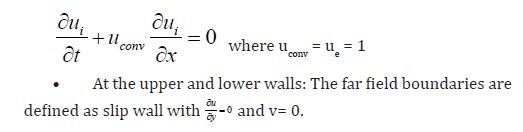#### Numerical Method

The dimensionless Navier-Stokes equations were numerically solved utilizing the following numerical technique based on the finite volume method . The temporal discretization of the time derivative is performed by a Euler backward second-order implicit scheme. Nonlinear terms are evaluated explicitly; then, viscous terms are treated implicitly. The strong velocity–pressure coupling present in the continuity and the momentum equations is handled by applying the projection method . A Poisson equation, with the divergence of the intermediate velocity field as the source term, is then computed to obtain the pressure correction and the real velocity field. A finite volume method was utilized on a staggered grid system in order to discretize the system of equations to be solved. Furthermore, the QUICK scheme of Hayase et al.  is applied to minimize the numerical diffusion for the advective terms. The discretized equations are computed utilizing the red and black point successive over-relaxation method  with the choice of optimum relaxation factors. Besides, the resolution of the Poisson equation is performed by applying an accelerated full multi-grid method .

The construction of the mesh is the first step in any numerical simulation. The used meshes in this investigation are based on a staggered grid where scalar quantities (pressure...) and located at the center of the cell, while, the velocity components are defined at the centers of faces of the volume controls.

In order to ensure accuracy, the grids have denser clustering at the vicinity of the barrier where strong gradients are expected. Conversely, away from the obstacle where the expected gradients are low, larger meshes are preferred.The generic variable ϕ stands for u, v or ϕ and m indicates the iteration time levels. In the above inequality, the subscript sequence (i, j) stand for the grid indexing in the x, and y directions respectively.

In conclusion, it is worth noting that computations were performed by applying a developed home code named “NASIM” (Navier Stokes Incompressible Multigrid) [31,32] utilizing finite volume method and the numerical procedure described above.

#### Results and Discussions

##### Time step independence test

To ensure the accuracy of the results, it is necessary to verify the numerical procedure.

The determination of the time step to be utilized in all calculations is very important, since, a too large time step size will yield inaccurate results while an excessively small-time step is computationally inefficient.

Furthermore, several time step refinements were performed for the present simulations with non-uniform grid m*n=768*160. It is worth noting that the grid independence study was conducted for two different non uniform grids, namely, m*n=560*340 and m*n=768*160. In the current investigation, independence of numerical results from the mesh size was assumed when the difference in the simulated values computed between two consecutive grids was less than 1%. It was concluded from the deviation values obtained that, a non-uniform grid of m*n=768*160 is sufficiently fine to ensure the grid independent solution and provides a good compromise between accuracy and CPU time in the range of variables to be investigated. Hence, the grid m*n=768*160 is applied to perform all subsequent calculations.

To ensure the temporal convergence of results, two Figures 3 and 4 are reported describing the temporal evolution of the lift coefficient and horizontal velocity components, respectively.

From Table 1, it is confirmed that the results of the calculations with the time step Δt = 0.001 are nearly identical to the results with Δt = 0.0025 and Δt = 0.0005. That’s why the time step Δt = 0.001 is applied in all simulations.

Table 1:Influence of time step (Δt) at Re = 50.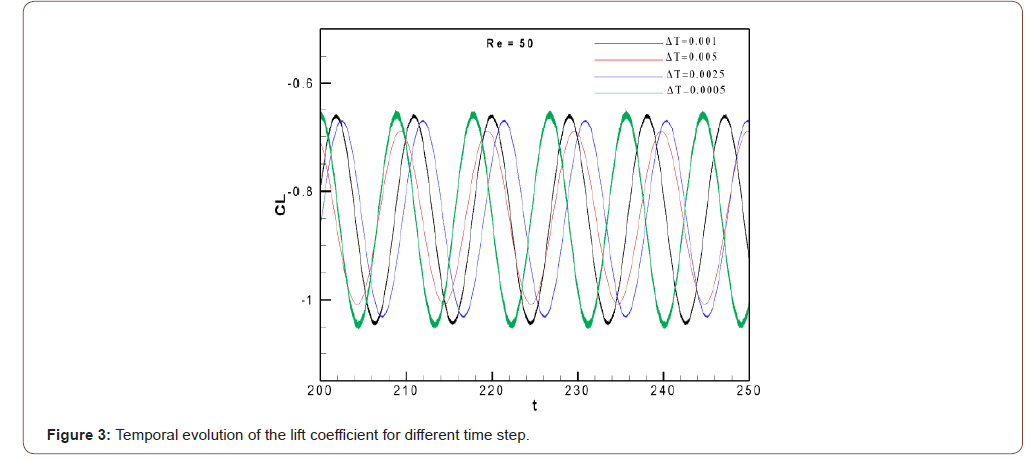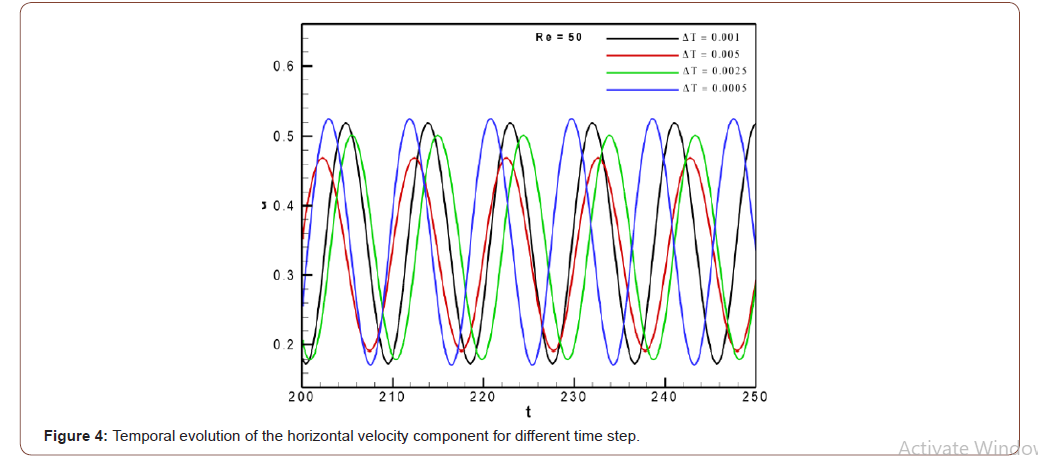##### Code validation

To give more confidence to the results of the current simulations, some quantitative and qualitative comparisons with other numerical investigations presented in the literature have been carried out.

The physical problem that is used for the validation of the inhouse code “NASIM” is the physical model investigated by Breuer et al. .

In the current study, validation of the simulations was carried out on a non-uniform mesh of dimension m*n =560*340 as the mesh used by Breuer et al. .

The study was conducted over a range of Reynolds number varying from 10 to 180 and analysis of the effect of this parameter on the evolution of the Strouhal number and lift and drag coefficients was carried out.

Then, the effect of Re on the Strouhal number (Figure 5) was performed. It is noted that for relatively low Reynolds numbers (50 < Re < 130) the Strouhal number increases with Re values. A significant change in the structure of flow takes place, namely the movement of separation point of the trailing edge to the leading edge of the square cylinder. The Strouhal number is at a maximum at nearly Re = 140 then decreases again for higher Reynolds numbers.

Figure 5 shows that our results are in good agreement with the results of Breuer et al.  and Galleti et al. .

As observed, this comparison validates our computer code making confidence on the presented results.

It is noted that validation of the computer code has already been presented by Fezai et al. [2,14,35-37] which is a contribution of the current investigation.

##### Influence of the type of triangular arrangement of cylinders on the bifurcation point from stationary to unsteady regime

After validation, attention is now focused to analyze the influence of the Reynolds number and the change of the triangular arrangement of the cylinders on the transition from steady to unsteady regime due to bifurcation. It can be seen in Figures 6,7 and 8 that there are two recirculating lobes occupying the entire space of the wake of each arrangement and the flow condition are steady. This is due to the fact that at such low value of Reynolds number the viscous forces are dominant and resist the movement of flow.

The temporal evolution of the horizontal velocity component U and vertical velocity component V relative to the two arrangements considered in this investigation are shown in Figures 7 and 9. From these figures, it can be observed that the amplitude of the oscillations increases and then, after a certain time, it decreases and tends towards zero, which means that the stationary flow is stable because all the disturbances are damped.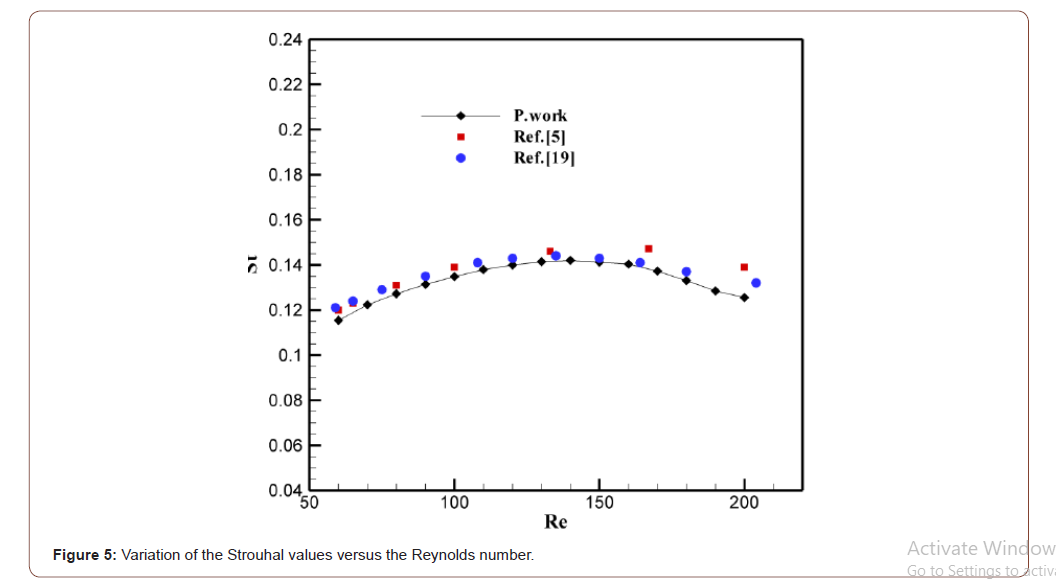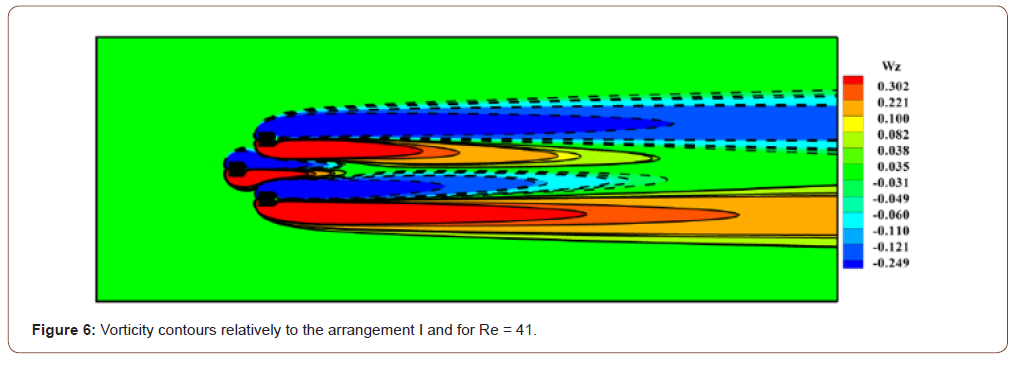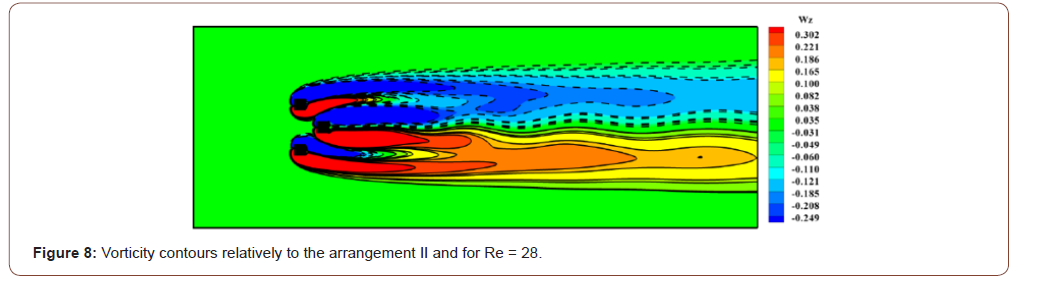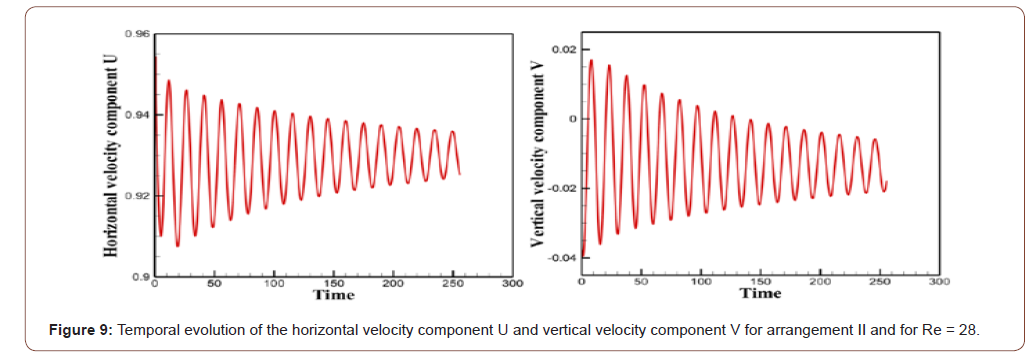It is very clear that the type of the triangular arrangement has ability to change flow behavior for different flow situations.

In fact, considering the first arrangement, the steady regime is observed for Re = 41 while it remains steady up to a Reynolds value equal to 28 relatively to the second arrangement. It can be deduced then that the first arrangement tends to stabilize the flow and strengthen the critical Reynolds value compared to the arrangement II in which the critical Reynolds number is very close to 28.

We can then deduce that the flow remains steady in the range 1≤Re≤41 for the arrangement I. For the cases of the arrangement II, the flow remains steady in the ranges 1≤ Re ≤ 28.

From this observation, it can be clinched that Rec>41 for the first arrangement and Rec>28 for the 2nd triangular arrangement.

As a result, the critical Re value at which the stationary character disappears depends strongly on the type of triangular arrangement of the three cylinders.

By increasing the Reynolds number, we note that the state of flow of the two triangular arrangements becomes unsteady and this is well visualized in Figures 10, 11, 12 and 13. It is clear that the perturbations can no longer be damped because the flow becomes unsteady and the Reynolds number exceeds the critical value.

Figures also shows that the unsteady state of flow occurs at a Reynolds number very close to 46.5 which means that the transitional state of flow around the first triangular arrangement remains in the range 41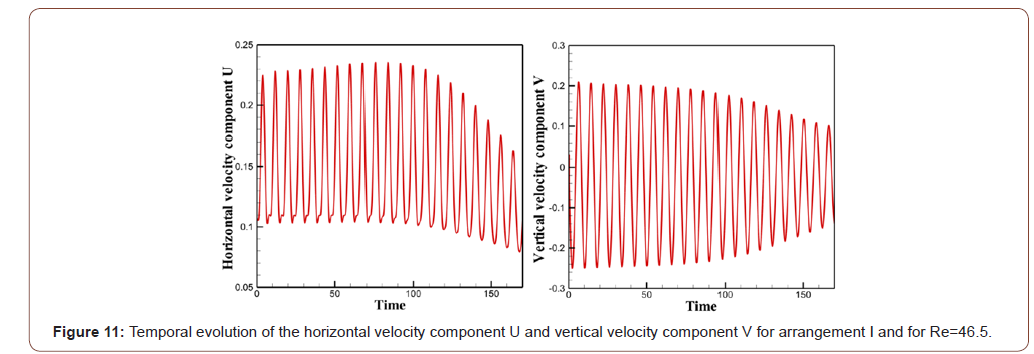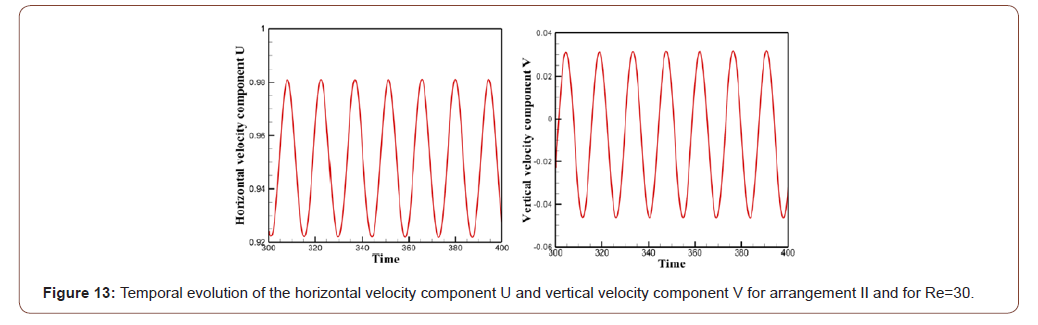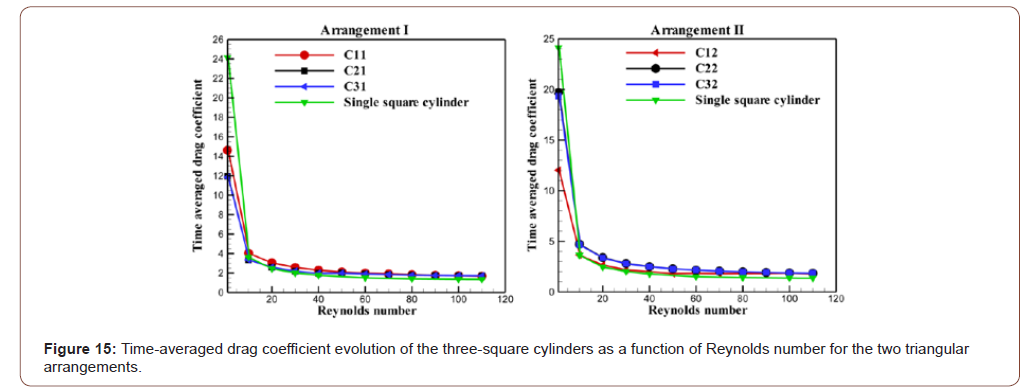We then find that the transition to the unsteady regime begins in the wake of the second triangular arrangement and then in the wake of the first arrangement.

Therefore, the two triangular arrangements of the three-square cylinders considered in this investigation result a significant reduction in the value of the critical Reynolds number. In addition, these triangular arrangements accelerate the birth and generation of vortices.

Comparing the present results with the one in which the obstacle is a single square cylinder, we note that there is a big difference in the flow structure. Again, the flow around a square cylinder remains stationary up to a fairly high Reynolds number compared to our results.

Indeed, Kelkar and Patankar  have focused on the study of instability that causes a steady laminar flow behind a square cylinder to result in an unstable laminar flow. They showed that the point of instability is between Re = 50 and 60 and they computed the value of the critical Reynolds number having a value Rec=53.

In the case of a flow around two square cylinders that are placed in line with a fixed space ratio g = 3.5 (g= spacing ratio) (Abbasi and Islam ), the unsteady regime appears when the Reynolds number has a value of 55.

Sarwar Abbasi et al.  also found that at g = 5 the unsteady state of flow occurs at Re = 85 which means that the transitional state of flow around four-inline cylinders remains in the range 68 ≤ Re ≤ 84 and the range of Re for transitional flow state in the cases of two- and three-inline cylinders is 63 ≤ Re ≤ 72 and 68 ≤ Re ≤ 82, respectively.

##### Influence of the type of the triangular arrangement of three-square cylinders on Strouhal number

The Strouhal number St as a function of Re for the two triangular arrangements considered is shown in Figure 14. It is very important to mention here that in the present investigation St indicates the primary vortex shedding frequency, while, itis calculated by applying fast Fourier transform (FFT) technique on lift coefficients.

From Figure 14, it can be observed that St is generated at Re = 50 in the arrangement I. However, in the arrangement II St is generated at Re = 30. It is important to mention here that the unsteady regime begins in arrangement II then in arrangement I and this comes back to the influence of the type of the triangular arrangement of the three cylinders. It can be seen that the St of the three cylinders increases monotonically with Re and this is well noted in the two arrangements (I and II). Furthermore, it exhibits increasing behavior with increment in the Re values. This is due to the fact that as Re increases, the shear layers become thin and the strength of vortices increases, which results in increment in the values of St.

It is clear from the figure that the values of the Strouhal number of the three cylinders in arrangement I are greater than the values of St of a single square cylinder. Inversely to arrangement I, the St values of the three cylinders in arrangement II are smaller than the St values of a single square cylinder.

Consequently, we can explain the increase and the decrease of the values of St which were noted respectively in arrangement I and arrangement II by the significant influence of the type of the triangular arrangement of the three cylinders on the mechanism of detachment of vortices.

We can also be seen in Figure 15 that the values St of the three cylinders in arrangement I are very close. Also, in arrangement II, the three cylinders have values very close to St. This indicates that all the cylinders lose vortices at very close frequencies.

##### Influence of the type of the triangular arrangement of the three-square cylinders on the drag force

According to previous studies , the addition of one or more squares cylinders in the wake of single body significantly affects the aerodynamic forces. We have also analyzed this phenomenon in current investigation.

The fluctuation of the average drag coefficient for flow around three square cylinders in two different triangular arrangements with Re is shown in Figure 16. For small Reynolds numbers, the average drag coefficient of all cylinders in both triangular arrangements show decreasing trend with increment in Re. The drag force induced on cylinders is developed by the pressure and viscous forces acting on the three cylinders. Therefore, the reason of this decrease is that as Re increases, the viscous forces become weaker. Due to this fact, the thickness of shear layers reduces, which results in weaker drag forces. Also, the average drag coefficient is higher in steady state of flow than that in transitional and unsteady states of flow because the CD values in these two states vary very slightly. In addition, it should be noted that the CD values of the three cylinders in the two triangular arrangements became almost equal when 60≤Re≤120. However, the difference between the CD values appears for the low Reynolds numbers.

Interestingly, the present results show that the CD values of the three cylinders in arrangement I are very low compared to those of arrangement II.

A comparison was made between the mean drag coefficient values of the three cylinders of each triangular arrangement and the CD values of an isolated square cylinder (Figure 15), it was found that the type of triangular arrangement of the three cylinders significantly changes the fluctuation in drag force.

For the low Reynolds numbers (1≤Re≤10), the CD values of the three cylinders in both arrangements are very low compared to those of the isolated cylinder. It can be deduced that the two triangular arrangements of the three cylinders result in a significant decrease in CD values. When 10≤Re≤50, for arrangement I the CD values of the two cylinders C21 and C31 are equal to the CD values of the isolated cylinder, but the CD values of the C11 cylinder are slightly higher compared to those of the isolated square cylinder. Inversely to arrangement I, all the CD values of the two cylinders C22 and C32 of arrangement II are found above the line for that of the single cylinder when 10≤Re≤50 and the CD curve of the C12 cylinder is found confused with that of the insulated square cylinder. When 50≤Re≤120, all curves of the average drag coefficient of the three cylinders in the two triangular arrangements are found above the line corresponding to the single square cylinder with a slight difference.

##### Influence of the type of the triangular arrangement of the three-square cylinders on the average lift coefficient

When a square cylinder is in cross flow, the lift force is only generated by vortex shedding caused by the movement of the vortex from top to bottom and from bottom to top. The dependency of mean lift coefficient is demonstrated in Figure 16. The single square cylinder data is also given for comparison.

Interestingly, the present results show that the values of the average lift coefficient of the two cylinders C2 and C3 evolve symmetrically with respect to the cylinder C1. Therefore, the mean lift coefficient of cylinder C2 has the identical magnitude but opposite sign as compared to that of cylinder C3 and this is well noted in the two triangular arrangements. The average lift coefficients of cylinder C3 are always negative, while those of cylinder C2 are positive, implying that lift forces on the cylinders are always repulsive. Again, for Arrangement I, it is found that there is a significant difference for mean lift coefficients between cylinders C2 and C3 for low Reynolds numbers because the largest value of CL corresponds to cylinder C3 for Re = 1 and the most low value of CL corresponds to cylinder C2 and also when Re = 1. By increasing the Reynolds number, we notice that the values of CL of cylinder C3 decrease and the values of CL of cylinder C2 increase. When 40

However, for arrangement II, the curve which corresponds to the third cylinder C3 increases with the increase in Reynolds numbers, so this increase is very important for the low Reynolds numbers (1

Similarly, for large values of the Reynolds number, the curves of cylinders C2 and C3 evolve symmetrically with respect to the curve of cylinder C1. This symmetry indicates that the direction of the lift force applied to the cylinder C2 is opposite to the direction of the lift force of the cylinder C3. Therefore, this inversely in the direction of the lift force is due to the arrangement of the three cylinders .

In general, the cylinder C32 has a CL greater than the other cylinders in the two arrangements considered in this study; however, cylinder C22 has the lowest CL values compared to the other cylinders in the two triangular arrangements. This might be due to the strong vortex shedding from the free stream sides. We can see that the type of triangular arrangement of the three cylinders has a considerable influence on the fluctuation of the lift force.

#### Conclusion

A numerical investigation was carried out to analyze the influence of the type of the triangular arrangement of the three square cylinders on the structure of a two-dimensional flow and on the aerodynamic forces using a numerical code based on a formulation of type finite volumes, the projection method and multi-grid acceleration.

For this study, Re was varied from 1 to 110. The results were presented in terms of vorticity contours, temporal evolution of velocity and temporal histories of drag and lift coefficients.

Important findings of this numerical investigation are given below:

• The effect of the type of the triangular arrangement of the three-square cylinders on the birth of the vortex detachment and on the transitional state from the steady state to the unsteady state was examined. We then found that the flow remains steady in the range 1≤Re≤41 for the arrangement I. For the cases of the arrangement II, the flow remains steady in the ranges 1≤ Re ≤ 28. Therefore, it can be clinched that Rec>41 for the first arrangement and Rec>28 for the 2nd triangular arrangement. It is very important to mention that the critical Re value depends strongly on the type of triangular arrangement of the three-square cylinders.

• The influence of the type of triangular arrangement on the evolution of the Strouhal number has been analyzed. The values of the Strouhal number of the three cylinders in arrangement I have been found to be greater than the values of St in arrangement II. This comes back to the significant influence of the type of triangular arrangement of the three cylinders on the detachment mechanism of the vortices.

• The fluctuation of the average drag coefficient for flow around three square cylinders in two different triangular arrangements with Re has been analyzed. Therefore, the CD values of the three cylinders in arrangement I are very low compared to those of arrangement II. It was found that the type of triangular arrangement of the three cylinders significantly changes the fluctuation in drag force.

• The purpose of the research is to know the effect of the type of the triangular arrangement of the three cylinders on the fluctuation of the lift; we have illustrated the variation of the average lift coefficient as a function of the Reynolds number. We have found that the two arrangements considered in this study considerably affect the fluctuation of the lift force.

None.

#### Conflict of Interest

No conflict of interest.

Article Details
Citation
Keywords
Scroll to
Scroll to Top